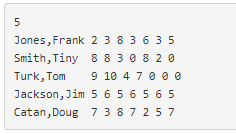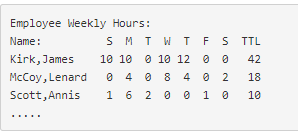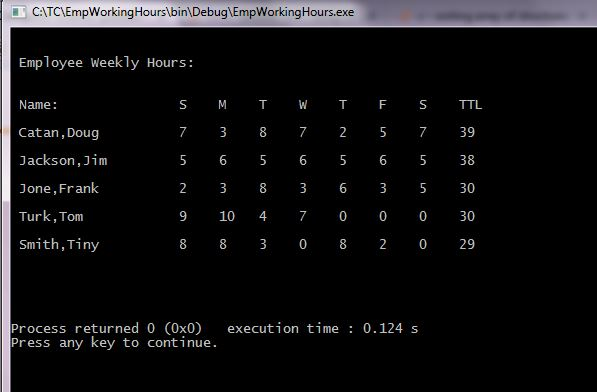# Question & Answer: of employee data for how many hours each employee worked for a week. Then for eac…..

Write a C++ program that will need to read from a file of employee data for how many hours each employee worked for a week. Then for each of the employees, a total of the hours worked is made and then the employees are sorted into descending order based on their total hours using bubble sort.

There needs be 3 function calls total in main that:

Don't use plagiarized sources. Get Your Custom Essay on
Question & Answer: of employee data for how many hours each employee worked for a week. Then for eac…..
GET AN ESSAY WRITTEN FOR YOU FROM AS LOW AS \$13/PAGE

Open and read the file into the array and add the total hours worked for each employee

Sort the array based on the total hours

Write out the output

Input file looks like this:Output should look something similar to this:Jones,Frank 2 3 8 3635 Smith, Tiny 883082 Turk, Tom 9104700 e Jackson,Jim 5 6 56 5 6 5 Catan,Doug 7 387257

C++ Program:

#include <iostream>
#include <iomanip>
#include <fstream>
#include <string>

using namespace std;

//Structure definition
struct Employee
{
string name;
int sun, mon, tue, wed, thu, fri, sat, TT;
};

//Function that reads data from file
void readData(fstream &fin, Employee employees[], int numEmp)
{
//Temporary object
Employee temp;

for(int i=0; i<numEmp; i++)
{
fin >> temp.name >> temp.sun >> temp.mon >> temp.tue >> temp.wed >> temp.thu >> temp.fri >> temp.sat;

temp.TT = temp.mon + temp.tue + temp.wed + temp.thu + temp.fri + temp.sat + temp.sun;

employees[i] = temp;
}
}

//Function that sorts data in descending order
void sortData(Employee employees[], int numEmp)
{
int i, j;

//Temporary object
Employee temp;

for(i=0; i<numEmp-1; i++)
{
//Iterating over remaining elements
for(j=0; j<numEmp-i-1; j++)
{
//Comparing total time
if(employees[j+1].TT > employees[j].TT)
{
//Swapping elements
temp = employees[j];
employees[j] = employees[j+1];
employees[j+1] = temp;

}
}
}
}

//Function that prints results
void writeReport(Employee employees[], int numEmp)
{
cout << “nn Employee Weekly Hours: n”;

cout << “nn ” << left << setw(20) << “Name:” << left << setw(5) << “S” << left << setw(5) << “M” << left << setw(5) << “T” << left << setw(5) << “W” << left << setw(5) << “T” << left << setw(5) << “F” << left << setw(5) << “S” << left << setw(10) << “TTL” << ” n”;

for(int i=0; i<numEmp; i++)
{
cout << “n ” << left << setw(20) << employees[i].name << left << setw(5) << employees[i].sun << left << setw(5) << employees[i].mon << left << setw(5) << employees[i].tue << left << setw(5) << employees[i].wed << left << setw(5) << employees[i].thu << left << setw(5) << employees[i].fri << left << setw(5) << employees[i].sat << left << setw(10) << employees[i].TT << ” n”;
}

cout << “nn”;
}

//Main function
int main()
{
int numEmp;

//Array of employees
Employee *employees;

fstream fin(“employees.txt”, ios::in);

//Checking file status
if(fin.fail())
{
cout << “nn Error reading file… nn”;
return -1;
}

//Reading number of records from file
fin >> numEmp;

//Allocating memory
employees = new Employee[numEmp];

//Closing file
fin.close();

//Sort data
sortData(employees, numEmp);

//Writing results
writeReport(employees, numEmp);

cout << “nn”;
return 0;
}

______________________________________________________________________________________

Sample Output: